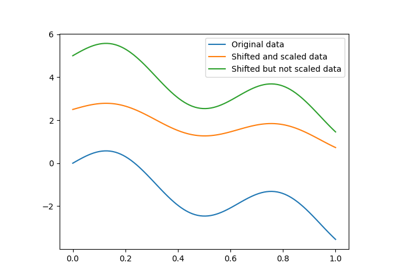gemseo / mlearning / transform

# pipeline module¶

A pipeline to chain transformers.

The Pipeline class chains a sequence of tranformers, and provides global fit(), transform(), fit_transform() and inverse_transform() methods.

class gemseo.mlearning.transform.pipeline.Pipeline(name='Pipeline', transformers=None)[source]

Bases: Transformer

Transformer pipeline.

Parameters:
• name (str) –

A name for this pipeline.

By default it is set to “Pipeline”.

• transformers (Sequence[Transformer]) – A sequence of transformers to be chained. The transformers are chained in the order of appearance in the list, i.e. the first transformer is applied first. If transformers is an empty list or None, then the pipeline transformer behaves like an identity transformer.

compute_jacobian(data)[source]

Compute the Jacobian of the pipeline.transform().

Parameters:

data (ndarray) – The data where the Jacobian is to be computed.

Returns:

The Jacobian matrix.

Return type:

ndarray

compute_jacobian_inverse(data)[source]

Compute the Jacobian of the pipeline.inverse_transform().

Parameters:

data (ndarray) – The data where the Jacobian is to be computed.

Returns:

The Jacobian matrix.

Return type:

ndarray

duplicate()[source]

Duplicate the current object.

Returns:

A deepcopy of the current instance.

Return type:

Pipeline

fit(data, *args)

Fit the transformer to the data.

Parameters:
Return type:

NoReturn

fit_transform(data, *args)

Fit the transformer to the data and transform the data.

Parameters:
Returns:

The transformed data.

Return type:

ndarray

inverse_transform(data)[source]

Perform an inverse transform on the data.

The data is inverse transformed sequentially, starting with the last transformer in the list.

Parameters:

data (ndarray) – The data to be inverse transformed.

Returns:

The inverse transformed data.

Return type:

ndarray

transform(data)[source]

Transform the data.

The data is transformed sequentially, where the output of one transformer is the input of the next.

Parameters:

data (ndarray) – The data to be transformed.

Returns:

The transformed data.

Return type:

ndarray

CROSSED = False
name: str

The name of the transformer.

parameters: str

The parameters of the transformer.

transformers: Sequence[Transformer]

The sequence of transformers.

## Examples using Pipeline¶Transformer pipeline example

Transformer pipeline example